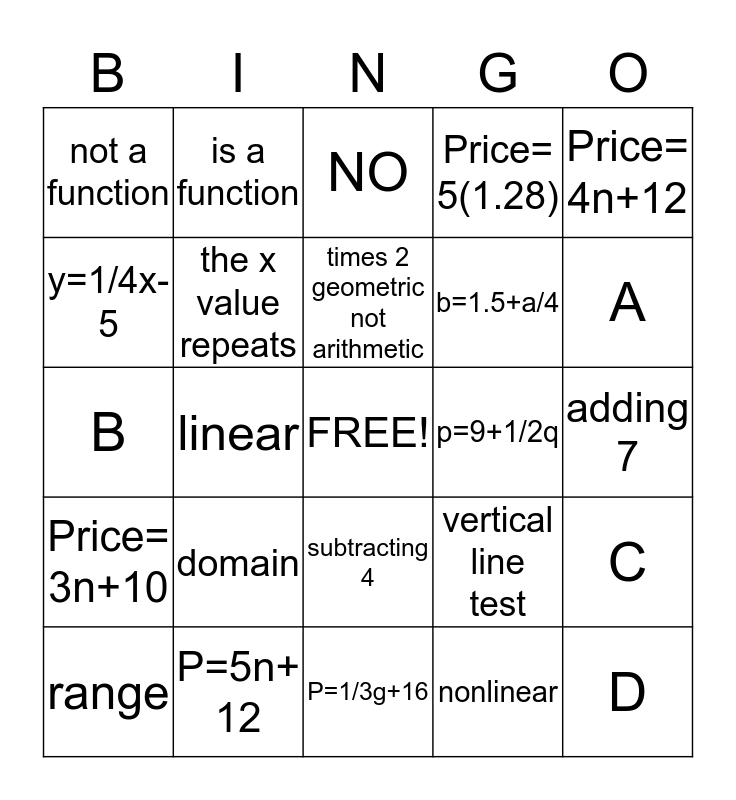# Algebra I-A Chapter 4 Test ReviewThis bingo card has a free space and 24 words: domain, P=5n+ 12, times 2 geometric not arithmetic, range, Price= 4n+12, not a function, y=1/4x-5, is a function, p=9+1/2q, subtracting 4, b=1.5+a/4, B, vertical line test, C, the x value repeats, nonlinear, adding 7, NO, A, linear, D, Price= 3n+10, Price= 5(1.28) and P=1/3g+16.

## Play Online